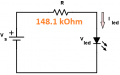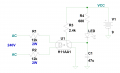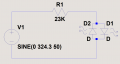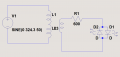# Most efficient design to make an LED AC indicator?

#### rambomhtri

Joined Nov 9, 2015
549
Hi, I was repairing this power strip that had a switch and a LED indicator. One of the problems was that the LED light was very dimmed. The resistor value is 148.1 kOhm, tested with my DMM and the color codes checks, and the LED specs... I can't tell, but I am assuming it's a 2V/10mA LED. Feel free to correct me during the whole process.

The scheme was like this:Since the impedance is purely resistive, then I guess we can do:
148100 Ohm * 0.01 A = 1481 V in the resistor

There is the first problem. The current would not follow a sine wave, because the LED would cut the wave during the negative cycle, so I guess the RMS would be 0.01 A / 2 = 0.005 A. Anyways, during the positive cycle it would have those 0.01 A value.

How is that going to be 1481 V?
Then the LED would have an opposite V and the LED would be generating energy because the current and voltage are opposed. Something's wrong. I am guessing that either the 2V or 10mA values I am assuming for the LED are not the values I should use.

The second problem: the efficiency of that design is so bad. You want to use an LED that is about 10-50mW and you design something to use that LED while consuming 1-3W in the process? So stupid.

So I basically wanted to redesign that so the LED lights up and is consuming like 80-90% of the whole circuit. I guess I will have to use caps and more components.

#### ericgibbs

Joined Jan 29, 2010
18,234
hi,
What is the Value of the Vs voltage.?

E

#### Alec_t

Joined Sep 17, 2013
14,009
Are you sure it's an LED and not a neon?

•rambomhtri and LesJones

#### Alec_t

Joined Sep 17, 2013
14,009
Yup. That's a LED. Unusual for a LED to dim noticeably, unless it's been energised for > 50000 hours or so.

#### rambomhtri

Joined Nov 9, 2015
549
So... how would you design the circuit?
How can I check the LED values?

I mean, I am willing to buy all the components, including the LED.

#### ericgibbs

Joined Jan 29, 2010
18,234
Hi
What is the measured Vsupply voltage.????
E

•BobaMosfet

#### rambomhtri

Joined Nov 9, 2015
549
Europe, 230V AC 50Hz

I would love to see your design, draw it and analyze it. After discussing it, I will build it.

#### ericgibbs

Joined Jan 29, 2010
18,234
hi rambo,
If your figures are correct, this shows why it could be a very low light level,
E

Last edited:

#### BobTPH

Joined Jun 5, 2013
8,108
Are you sure that circuit is correct? LEDs can withstand only 6V when reverse biased. This circuit would put a peak of 315V in reverse!

I am surprised that it did not fail immediately. It might have degraded the LED over time.

Bob

#### Dave Lowther

Joined Sep 8, 2016
205
Since the impedance is purely resistive, then I guess we can do:
148100 Ohm * 0.01 A = 1481 V in the resistor
That's not how it works. The unknown is current, you know the voltage and the resistance.
Ignoring the LED for the moment, if you just had the resistor wired across the 230V (rms) then you would have a current through the resistor of 230/148100 = about 1.5 mA (rms)
Considering the LED now, to a first approximation we can ignore the 2V approx forward voltage across the LED.
If the rms of a 1/2 wave rectified sine wave is 1/2 the rms of the original sine wave then you will have a current of about 0.75 mA (rms) through the resistor and the diode. The power dissipated by the resistor will be about 90 mW.

I don't think LEDs have a high enough reverse breakdown voltage to be connected as shown in your diagram. I would have expected at least a regular diode across the LED to conduct on the opposite half cycle that the LED conducts on. That would double the power dissipated by the resistor.
 I was writing the above while the previous two posts above were posted, so there's some duplication of info in my post

#### BobaMosfet

Joined Jul 1, 2009
2,102
Most LEDs can withstand very little reverse current- they don't have the strength of an actual diode. If you want to make it solid bright, you need to use a full-wave bridge rectifier, capacitors, and resistors. Because it's related to MAINS, I'd use Safety Capacitors- guaranteed to fail open, not shorted.

Bridge Rectifier: 3N257 Use something like this. 420VAC RMS at up to 2A. Based on frequency, you can drop the voltage and current using Safety Capacitors beforehand, if you want to use a smaller rectifier (like a W02G, for example).

Last edited:

#### eetech00

Joined Jun 8, 2013
3,653
Hi, I was repairing this power strip that had a switch and a LED indicator. One of the problems was that the LED light was very dimmed. The resistor value is 148.1 kOhm, tested with my DMM and the color codes checks, and the LED specs... I can't tell, but I am assuming it's a 2V/10mA LED. Feel free to correct me during the whole process.

The scheme was like this:
View attachment 242464

Since the impedance is purely resistive, then I guess we can do:
148100 Ohm * 0.01 A = 1481 V in the resistor

There is the first problem. The current would not follow a sine wave, because the LED would cut the wave during the negative cycle, so I guess the RMS would be 0.01 A / 2 = 0.005 A. Anyways, during the positive cycle it would have those 0.01 A value.

How is that going to be 1481 V?
Then the LED would have an opposite V and the LED would be generating energy because the current and voltage are opposed. Something's wrong. I am guessing that either the 2V or 10mA values I am assuming for the LED are not the values I should use.

The second problem: the efficiency of that design is so bad. You want to use an LED that is about 10-50mW and you design something to use that LED while consuming 1-3W in the process? So stupid.

So I basically wanted to redesign that so the LED lights up and is consuming like 80-90% of the whole circuit. I guess I will have to use caps and more components.
Do you have a DC power supply?
Can you remove the LED from the AC circuit for testing?

if so, you can find out the LED Vf and If relationship you need to know to calculate the correct component values.

#### Dave Lowther

Joined Sep 8, 2016
205
find out the LED Vf and If relationship you need to know to calculate the correct component values.
Isn't Vf insignificant? My rationale for thinking so is that any Vf value between 0V and 10V would make < 5% difference to the voltage across the resistor. It's essentially the resistor value that defines the forward current independently of the LED Vf. Although I can see that measuring the current at the desired brightness would enable choice of component values.
However, choice of current is going to have a big influence on the power rating of the resistor. For example, at 10mA full wave rectification the resistor would dissipate 2.3W and should probably be one rated at 5W.
Edit: I'm not ruling out the capacitor solution, I was just limiting the scope of the solution ATM to match the TS's solution.

#### eetech00

Joined Jun 8, 2013
3,653
Isn't Vf insignificant? My rationale for thinking so is that any Vf value between 0V and 10V would make < 5% difference to the voltage across the resistor. It's essentially the resistor value that defines the forward current independently of the LED Vf
Vf for an LED (or diode) is always significant (IMO). It is true that the resistor "limits If" the current flow, but it is not independent from Vf. The device will not conduct current until a specific voltage (Vf) is developed across its terminals and Vf is reflected as the "voltage drop" across it. How much the voltage drop "matters" depends on the circuit.

I would use the output of an AC Opto Isolator to drive the LED.Last edited:

#### BobTPH

Joined Jun 5, 2013
8,108
It is insignificant in the calculation when the supply voltage is 315V. The equation is

If = (V - Vf) / R

When Vf is less than 3V, it can change the current by at most t 1%.

Bob

#### Ian0

Joined Aug 7, 2020
8,947
The most efficient LED indicator possible must be to use a dual-chip LED (with two LEDs in anti-parallel) and drive it with a capacitor. (68nF for 5mA through the LED from a 230V supply)
However, it will tend to fail on start-up transients - but the quest was for the most efficient, not the most reliable.
Adding resistance in series will make it more reliable but less efficient.
(But I'd use a neon)

#### dcbingaman

Joined Jun 30, 2021
1,065
So... how would you design the circuit?
How can I check the LED values?

I mean, I am willing to buy all the components, including the LED.
If you want 10mA average that is 230V/0.01A = 23K Resistor
I would recommend two LED's in parallel but with opposite polarity so you get brightness onboth ends of the AC Cycle and avoid blowing up either one due to reverse bias voltage to high.
The 23K resistor power is .01^2*23K = 2.3W resistor. Recommend a minimum 10W resistor.
I ignored the LED drop as it is insignificant compared to 230Vrms.
For maximum brightness be sure to read your LED datasheet. If it is say 20mA, you are going to have to halve the resistor to about 11.5K but now the resistor will consume 4 times as much power (I^2*R) or close to 10 Watts! Then you need at least a 25W resistor.
I think you will find better circuits that don't waste so much power if you use instead a neon bulb and resistor.

Last edited:

#### dcbingaman

Joined Jun 30, 2021
1,065
Hi, I was repairing this power strip that had a switch and a LED indicator. One of the problems was that the LED light was very dimmed. The resistor value is 148.1 kOhm, tested with my DMM and the color codes checks, and the LED specs... I can't tell, but I am assuming it's a 2V/10mA LED. Feel free to correct me during the whole process.

The scheme was like this:
View attachment 242464

Since the impedance is purely resistive, then I guess we can do:
148100 Ohm * 0.01 A = 1481 V in the resistor

There is the first problem. The current would not follow a sine wave, because the LED would cut the wave during the negative cycle, so I guess the RMS would be 0.01 A / 2 = 0.005 A. Anyways, during the positive cycle it would have those 0.01 A value.

How is that going to be 1481 V?
Then the LED would have an opposite V and the LED would be generating energy because the current and voltage are opposed. Something's wrong. I am guessing that either the 2V or 10mA values I am assuming for the LED are not the values I should use.

The second problem: the efficiency of that design is so bad. You want to use an LED that is about 10-50mW and you design something to use that LED while consuming 1-3W in the process? So stupid.

So I basically wanted to redesign that so the LED lights up and is consuming like 80-90% of the whole circuit. I guess I will have to use caps and more components.
OOPS! Sorry I missed the part about lets do it efficiently! Here is a more efficient way:

Being that it is AC you can get away with a step down transformer to say 12VAC and drive the LED this way:Make it say around 20 to 1 secondary will be close to 11.5VAC D2/D1 will drop about 2V leaving you with 9.5V max on R1. Because each LED is only receiver power 1/2 of the time you could probably reduce R1 more being we only want the AVERAGE current through D1 and D2 being 20mA in this example. This will waste almost no power at all. Max power would be around 12^2/600 or 0.24Watts, R1 could be say a 1/2W resistor, there will be a little of power loss in the transformer, but most likely insignificant. In fact it will be less than that because the LED's don't conduct until they get to 2V so conduction time slightly less.

Last edited: